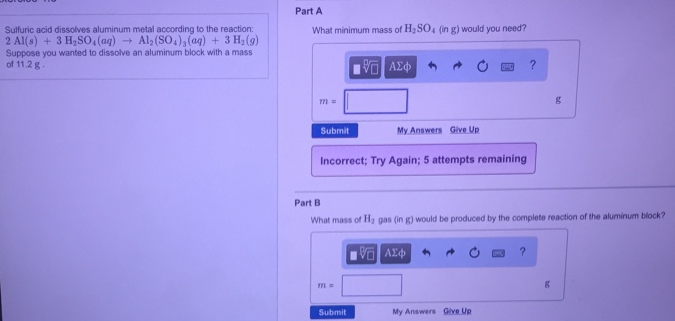# Problem: Sulfuric acid dissolves aluminum metal according to the reaction:2 Al(s) + 3H2SO4(aq) ---&gt; Al2(SO4)3(aq) + 3 H(g)Suppose you wanted to dissolve an aluminum block with a mass of 11.2 g. What minimum mass of H2SO4 (in g) would you need? What mass of H2, gas (in g) would be produced by the complete reaction of the aluminum block?

###### FREE Expert Solution
80% (301 ratings)###### Problem Details

Sulfuric acid dissolves aluminum metal according to the reaction:

2 Al(s) + 3H2SO4(aq) ---> Al2(SO4)3(aq) + 3 H(g)

Suppose you wanted to dissolve an aluminum block with a mass of 11.2 g. What minimum mass of H2SO4 (in g) would you need?

What mass of H2, gas (in g) would be produced by the complete reaction of the aluminum block?